Update January 10th 2012

Original paper presented at the MIXDES Conference 2011Burignat, S. & De Vos, A. "Test of a Majority-based Reversible (Quantum) 4 bits Ripple-carry Adder in Adiabatic Calculation", Proceedings of the 18th International Conference "Mixed Design of Integrated Circuits and Systems" (MIXDES~2011), 2011, pp. 368-373 BibTeX

Test of a majority-based reversible (quantum)
4 bits ripple-carry adder in adiabatic calculation

Stéphane Burignat and Alexis De Vos
Universiteit Gent,
Vakgroep elektronika en informatiesystemen
Sint Pietersnieuwstraat 41
B - 9000 Gent
Belgium
Email: research@burignat.eu; alex@elis.ugent.be

AbstractQuantum computing and circuits are of growing interestand so is reversible logic as it plays an important role in thesynthesis of circuits dedicated to quantum computation. Moreover,reversible logic provides an alternative to classical computingmachines, that may overcome many of the power dissipationproblems in the near future. As a proof of concept we designedand tested a reversible 4 bits ripple-carry adder based on ado-spy-undo structure. This paper presents some performancesobtained with such a chip processed in standard 0.35  μm CMOS technology and used in real reversible calculation (in this study,computations are performed in both directions such that additionand subtraction are made reversibly with the same chip). We alsodiscuss the superiority of using adiabatic signals over classicalrectangular pulses when using dual-line pass-transistor logicgates. Adiabatic signals allow the signal energy stored on thevarious capacitances of the circuit to be redistributed ratherthan being dissipated as heat. Finally, we show that adiabaticsignals allow to avoid calculation errors introduced by the useof conventional rectangular pulses and allow to drasticallyreduce the number of pulse resynchronization in large circuits.

Index Terms—reversible computation, design, implementation,pass-transistor logic, ripple-carry adder, Spectre simulation,quantum computation, adiabatic signal, test and measurement.

I. Introduction

Reversible computing is useful both in lossless classical computing   and in quantum computing  . Reversible circuits also present less power consumption against classical ones  .

The physical implementation of the adder we are presenting is reversible dual-line complementary pass-transistor CMOS logic   and does not make use of buffer of any sort nor level restorer. This adder has been extended and embedded in larger components such as a multiplier   and, more recently, in a H264/AVC encoder  .

We present for the very first time to our knowledge, extensive electrical tests performed on a reversible CMOS chip computing in both directions: forward (adder) and backward (subtractor) and compare the efficiency of both classical rectangular pulses and adiabatic signals.

II. Short history and description of the reversible adder chip

A. Short history

In 2005, Cuccaro et Al.   presented a new linear-depth ripple-carry quantum addition circuit making use only of controlled-NOT (CNOT or Feynman) gates and controlled-controlled-NOT (CCNOT or Toffoli) gates which was an improved version of a V-shaped reversible adder presented by  . In 2008,   presented the synthesis and design of a reversible Fourier transform making use of such a reversible adder, but using a do-spy-undo (majority-unmajority) scheme structure as firstly presented by  . This design was making use only of controlled-NOT (Feynman) gates and controlled-SWAP (Fredkin) gates1 . Unfortunately, this 8 bits adder was embedded in a larger 8 bits multiplier making it impossible specific access to measurements.

The circuit we present in this paper is a 4  bits majority-based reversible ripple-carry adder. As in  , it makes use only of Feynman and Fredkin gates and presents, as a general structure, a do-spy-undo scheme  . Full details about synthesis discussion, structure and theoretical consumption are already presented in  .

B. Block structure

As a summary, let us recall the block structure of the studied adder. Let n denote the size of both numbers to be added. First, as a do-spy-undo structure is used, each bit addition implemented, except the most significant bit (MSB), necessites one do-undo circuit. The 3 inputs majority do circuit and the 3 inputs unmajority undo circuit are presented in Fig.1 and Fig.2, respectively.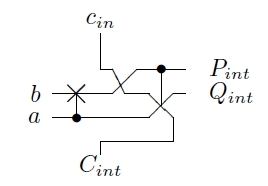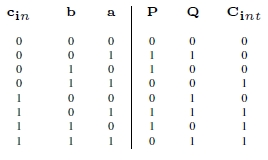Fig. 1.  Quantum diagram and truth table of a majority (do) block.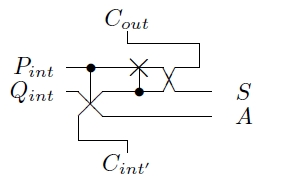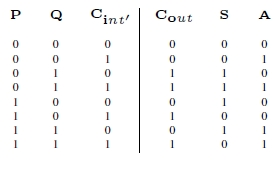Fig. 2.  Quantum diagram and truth table of an unmajority (undo) block

The do-undo block constitutes a one bit adder when used forward (respectively a one bit subtractor if used backward). The numbers a and b (respectively S and A) are the main inputs and Cin (Cout) is the carry-in. The number S (b) represents the computation result (sum a + b respectively difference S - A) and A (a) is a copy (garbage) of the input a (A) and so is the output Cout (Cin) with respect to the input Cin (Cout).

When an addition is computed (forward calculation), the internal bits Cint are used for carry transmission from one stage to the next during the majority operations, while the input Cint of the undo block rebuild the initial input cin during the undo operations (Cout = cin). When a difference is computed (backward calculation), the inverse process occurs leading to the calculation of the difference.

For 1 bit calculation, Cint would be directly connected to Cint whereas if the adder size n 2, the most significant bits addition is performed by using only two Feynman gates; one is used to compute the bit sum XOR () and the other to sum-up the carry to the final result. Each extra bit addition is realized by cascading supplementary do-undo blocks, linked together by connecting Cint to cin and Cout to Cint as presented in the full schematic of the 4 bits adder in Fig.3.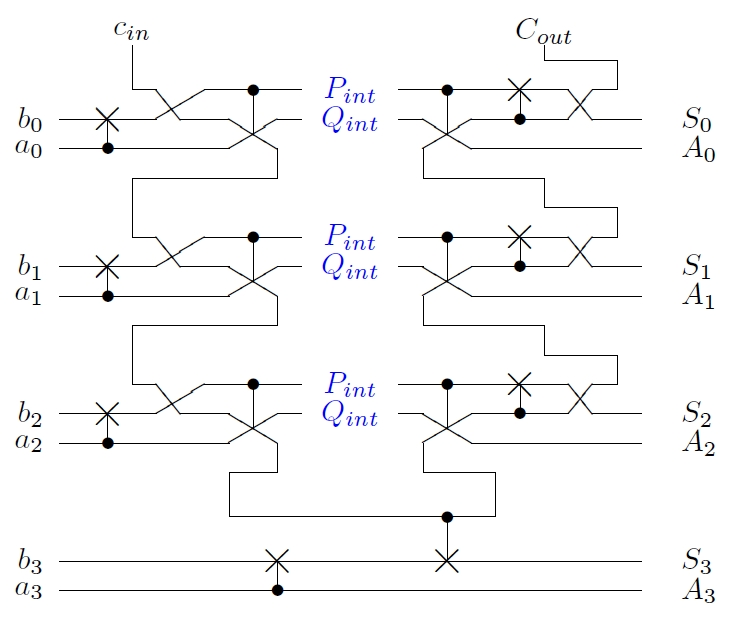Fig. 3.   Full quantum diagram of the 4 bits Cuccaro adder.

Let us notice that simplification presented in Fig.4 has been used for the real design of the unmajority block.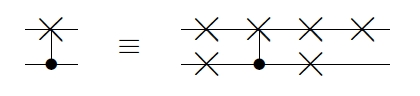Fig. 4.   Simplification sometime used for drawing of the real circuit.

III. Design and realization

This 4 bits Cuccaro adder has been designed using the Cadence© computer-aided design environment software. Each electrical simulation has been performed using its Cadence Spectre© simulator (see Fig.7 and Fig.8 as examples).

The chip processing has been realized at ONSemiconductor through the Europractice  / IMEC consortium in 350 nm standard CMOS technology. The transistor lengths used are L = 350  nm, both for n-type and p-type transistors, while widths are respectively Wn = 500  nm and Wp = 1500  nm. The chip contains a total of 160 transistors (80 n-type and 80 p-type).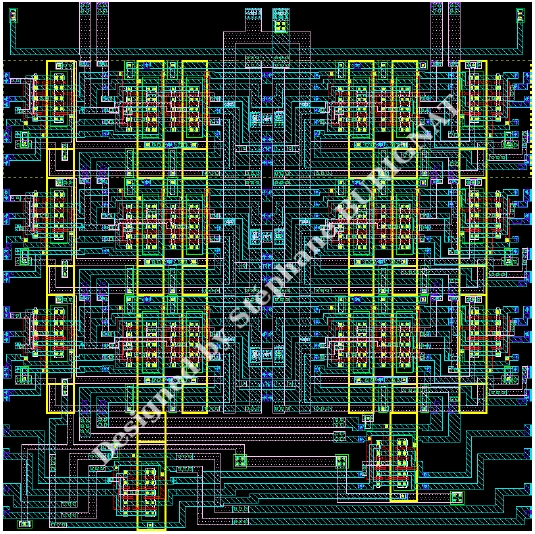Fig. 5.   Cadence Virtuoso© layout of the studied 4 bits Cuccaro adder.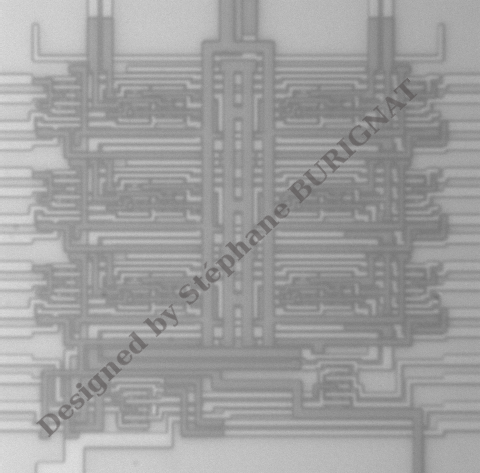Fig. 6.   Photograph of the processed 4 bits Cuccaro adder chip (90  μm x 90  μm ).

The Cadence Layout of the studied adder is shown in Fig.5 while Fig.6 presents a photograph of the realized chip (90  μm  x  90  μm). We can easily recognize the dual inputs and outputs at each left and right hand sides, and the two dual carries Cin and Cout at the top side while in the middle part are the wider wires used for the substrate and the N-wells biasing (typicaly, V SS = - 1 . 2  V and V DD = 1 . 2  V ).

IV. Simulation results

Starting with the simulation, two different types of signal have been used: ”traditional” square pulses and quasi-adiabatic triangle pulses.

A. Input signal definitions

The maximal signal voltages used, both for simulation and for measurements are V + = 1  V for logic ”1” and V - = - 1  V for logic ”0”.

If the first type of signal (square pulse) is applied, for the unchanged bits, the voltages corresponding to the desired logic levels remain constant while others are fastly switched. If the second signal type (adiabatic) is applied, a triangular pulse ranges from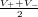to the desired logic level at each calculation step. Thus, in case of the second signal type, all signals (i.e. both the unchanged bits and the changing ones) temporarily come to the equilibrium voltage, half-way logic ”1” and logic ”0”. The two signal types can be seen in Fig.7 presenting the minuend S and subtrahends A of nine subsequent subtractions S - A.

B. Simulated outputs

Let us stress that the pulses of Fig.7 are representative of the input signals previously defined. Fig.8 presents the corresponding difference b and garbage a calculated outputs when using the Cuccaro adder in reverse mode (subtractor), for both the square and triangle adiabiatic pulse shapes. In Fig.8, we see that rectangular pulses do not all have the same amplitude, that they are also presenting large transition peaks while for the triangle adiabatic signals, the pulses are regular, without any transition peak.

Let us also notice that when the size n of the numbers to be computed is larger than two, some exceptional errors may occur when square signals are used while this doesn’t happen when adiabatic signals are applied (Fig.8 ).

One reason for that comes directly from the transmission gate that is build of two complementary transistors in parallel. Due to the steep slope generated during the transition of the square pulse, if a delay occurs between the control signals on the transistor gates and the signals transmitted through the transistor channels,the gates do not shut down the signal at the very moment of the transition and an opposite signal that would have been cut down is transmitted anyway, occasionally causing calculation errors.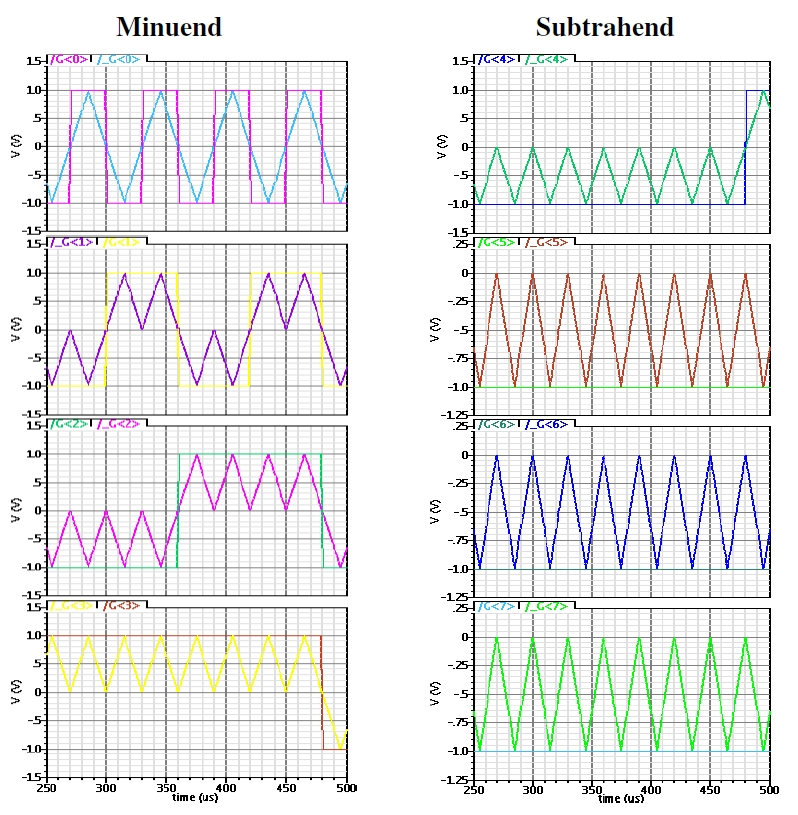Fig. 7.   Cadence Spectre© simulation: comparison of rectangular and adiabatic input pulses of same frequency.
Top line is the least significant bits
S0 and A0 whereas the bottom one corresponds to the most significant bits S3 and A3.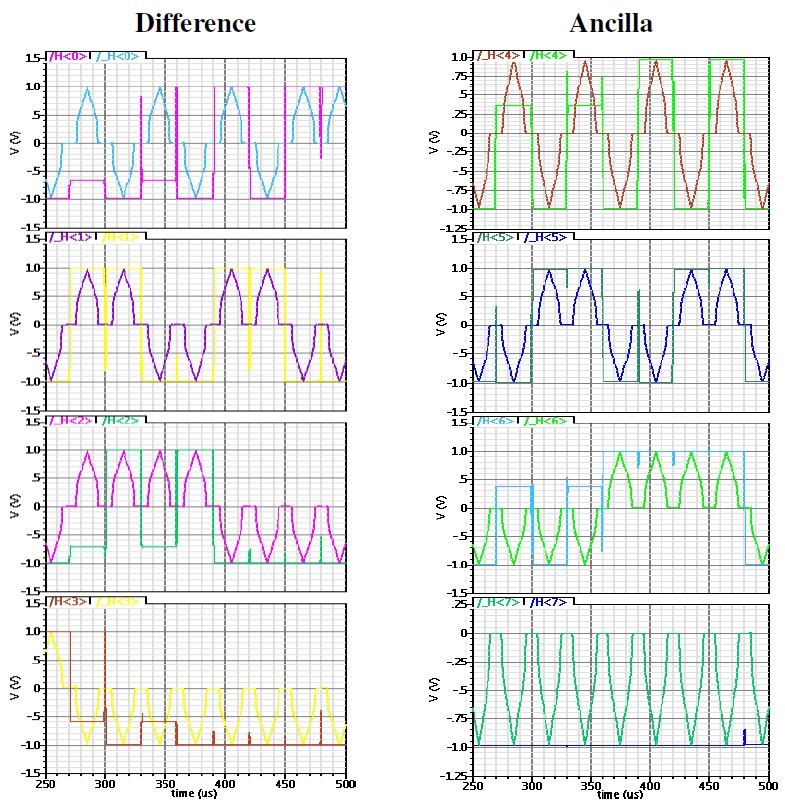Fig. 8.   Cadence Spectre© simulation: comparison of the output signals when using rectangular and adiabatic pulses, respectively.
According to Spectre
© electrical simulation software, using rectangular pulses introduces several errors in computation.
None of these errors occur when using triangular pulses.

Top line is the least significant b0 and a0 bit whereas the bottom one corresponds to the most significant ones b3 and a3.

If the adiabatic signals are used, the smooth transitions allow a delay between the signals. In effect, during the transition steps, the two transistors are working below their threshold voltages, drastically lowering the amplitude of the output signal (see also Fig.10 and comments). As the example of Fig.9 shows, a phase difference – expressed as a percentage of the period – as large as a quarter of a period PrcT = ± 25   % (plain lines) does not introduce any calculation errors, even if the final shape of the signal is deformed and if some small variations of the output potential (inferior to 40  mV ) appear when the transmission gate is closed. In fact, this maximal percentage will be dependant of the ratio between the maximum pulse voltage and the pseudo-threshold voltage of the transmisson-gates used. When a bigger phase appears, the output signal is no more zeroed before the command pulse.

A positive (respectively negative) phase indicates a delay of the input (respectively command) signal with respect to the command (respectively input) pulse 2.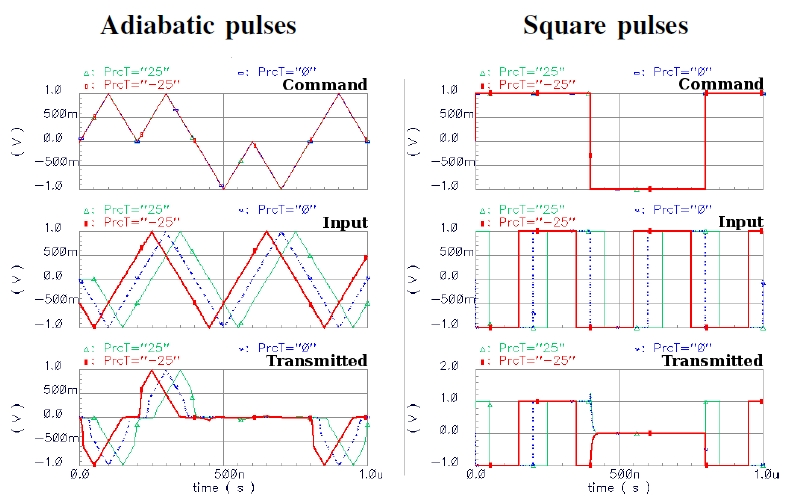Fig. 9.   Cadence Spectre© simulation of a complementary pass transistor gate: comparison between adiabatic and square pulses when a dephasing of PrcT = - 25% (bold) and 25% of the period is applied between the command pulses and the signal to be transmitted.
The dashed lines correspond
to a phase equal to 0   % . The frequency used is 5 MHz.

Further in the calculation steps, the signals may become symmetrical again, when two phased signals are used successively as the command and the transmitted signals. This then leads to a narrowing of the final pulse width (as seen in Fig.8) but does not impact significantly its maximal amplitude that is related to the impedance of the circuits. In Fig.9 (dash lines), the simulated output signal obtained from a single transmission gate when the phase equals zero (PrcT = 0   %) is larger than the output signals obtained from a complex circuit such as a subtractor (Fig.8), where a phase is introduced by the propagation of the internal carries Cint and Cint . In effect, in the Cuccaro adder, the cin signal when going through the whole computation, has to be transmitted twice at each stage of the calculation. The greater the bit size n, the greater the number of stages, the greater the phase introduced, the narrower the pulse. When using a square signal, this may produce wrong pulses whereas when using an adiabatic shape, the signal will be deformed, narrowed, but the calculation will remain possible with very few loss in amplitude and still guaranteeing a correct logic “1” or correct logic “0”. This was checked up to n = 6 bits.

It is also easy to verify that dual signals (e.g. a and a) are closely synchronized by symmetry of the dual circuits – each signal and its complementary having passed through the same number of transmission gates.

As a consequence, even if we need to pay attention to the design of the circuit in order to limit the phase introduced between the different signals used in the calculation, the triangular adiabatic shape signals allow to drastically reduce the number of level restorers and pulse resynchronizers.

V. Tests and measurements

Several extensive measurement steps have been performed on the Cuccaro circuit, both in forward (adder) and in reverse (subtractor) calculations.

Constant voltage computations are primary presented allowing to bring information principally on impedance and voltage bias impact on calculation. In a second hand, we present the adiabatic generator used for the experimental application of the input pulses. We then present measurements of the outputs obtained both in direct and reverse calculations.

A. Preliminary DC measurements

The voltages applied: substrate and NWELL biases, input constant biases, have been measured using a Flucke  175 multimeter in DC mode.

A large part of the truth table has been first successfully checked and some measurements have been done for some particular conditions:

• In direct calculation (adder), 32 different conditions: cin = 0, b = 1010 and a varying, as well as cin = 0, a = 0101 and b varying.
• In reverse calculation (subtraction), 16 different test vectors: (Cout = 0, A = 0101 and S varying).

From these measurements, the maximum voltage drop found for the adder is 30  mV whereas the maximum voltage drop for subtractor was found to be 6  mV over a constant input bias of 1  V . In any cases, the output drops are inferior to 3   % of the input signal biases which is the order of magnitude of the measurement incertainties.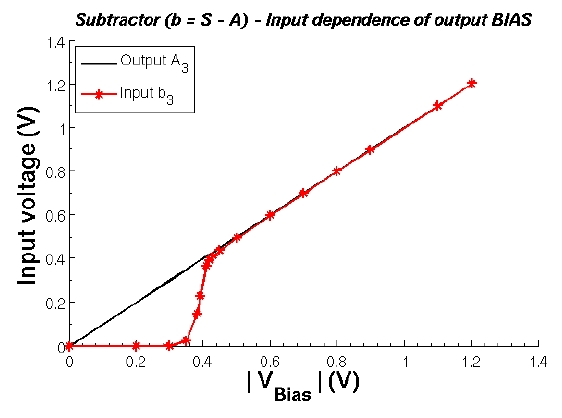Fig. 10.   Measurements of the input voltage dependance of bit b2 as a function of the output voltage bias of bit S2, for the reverse (subtractor) calculation:
b = S - A = 0110 - 0 1 01 = 0 0 01 .

Fig.10 presents a typical variation of an input voltage as a function of the output voltage bias when the adder is used in reverse mode (i.e. as subtractor). In fact, same curves are obtained for each output whether in direct mode (adder) or reverse one (subtractor), except for the most significant bit A3 (respectively a3) that is made by a direct connection to its corresponding input a3 (respectively A3) (see Fig.3). For all measurement performed, the voltages V DD and V SS = V GND are kept constant to 1 . 2  V and - 1 . 2  V respectively.

We see from Fig.10, that a minimal voltage input as small as 420  mV can still be used (without security marge) to perform calculations with this Cuccaro chip. This is also coherent with the general shape of simulated output pulses in Fig.8 and with the experimental measurements in Fig.12 and Fig.13 that present the same transition when applying a linear input variation (triangular adiabatic pulse). But in these last cases, one also needs to account for the impact of the phases introduced by the internal carry signals that not only narrow the pulse width but also slightly increase the minimal voltage input possibly used to perform a calculation. We then arbitrarily fix the limit of ± 500  mV as the minimum logical amplitude acceptable to define a logic ”1” (above 500  mV ) or a logic ”0” (below - 500  mV ). This choice is experimentally driven by the fact that within these two defined domains, the calculated signals obtained have always been found conform to what was expected.

The pseudo-threshold voltage3 V pT of the Cuccaro adder is found to be about 350  mV which is also close to the simulated value of the transmission-gate pseudo-threshold voltage. Between about 420  mV down to V pT = 350  mV a transition occurs that corresponds to the linear mode of the transmission gate transistors. Below V pT, the transmission gates are blocked and, as expected, no signal is transmitted.

B. Generation of the adiabatic signal

In order to apply the necessary adiabatic pulses, we designed an electronic module allowing to genetrate 4 types of triangle pulses:

1. A logic ”1” ranging from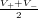up to +1  V and back,
2. A logic ”0” ranging from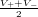down to - 1  V and back,
3. A switchig signal ranging between +1  V and - 1  V ,
4. The dual signal of the switching signal that is the symetrical signal from the mean value.

These four signals are all build up from one single triangular pulse ranging between +1  V and - 1  V by using a single buffer (switching pulse), an inverter followed by a buffer (dual switching pulse) or using a precision full-wave rectifier followed either by a buffer or by an inverter and a buffer (logic ”1” and logic ”0” respectively). Fig.11 presents the full schematic of the electronic module used to generate the experimental adiabatic pulses. It is a very simple thus very accurate circuit presenting very low output impedances. The operational amplifiers are polarized at ± 3  V while the input triangular signal has an amplitude of 2  V . This allows the operational amplifiers to work in the linear part of their characteristics and gives very accurate and reproducible pulses.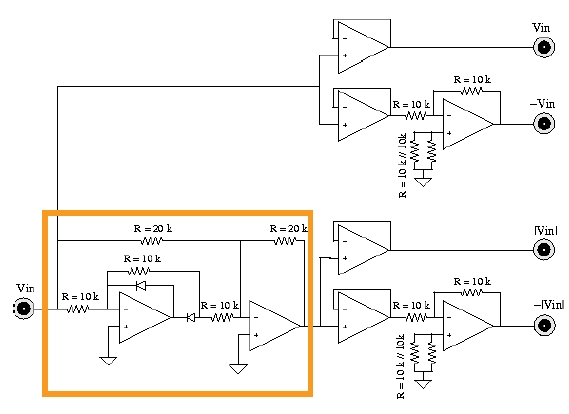Fig. 11.   Electronic schematic of the adiabatic signal generator module embedding an accurate full-wave rectifier (framed).

C. Adiabatic AC measurements

AC measurements have been performed using a commercial TektronicsTM TDS  210 digital oscilloscope. The output charge is its × 1 probe that can be modeled by a 1  M Ω resistor in paralell with a 32  pF capacitance.

By using the voltage divider technique, the experimental output impedance of the Cuccaro adder (or subtractor) is found to be Zout 5250   Ω ± 5   % whereas it is found to be close to 8  k Ω by Cadence Sperctre© simulation.

Fig.12 and Fig.13 show typical experimental measurements realized respectively in forward (adder) and backward (subtractor) computation.

Experimental Measurements in forward computation –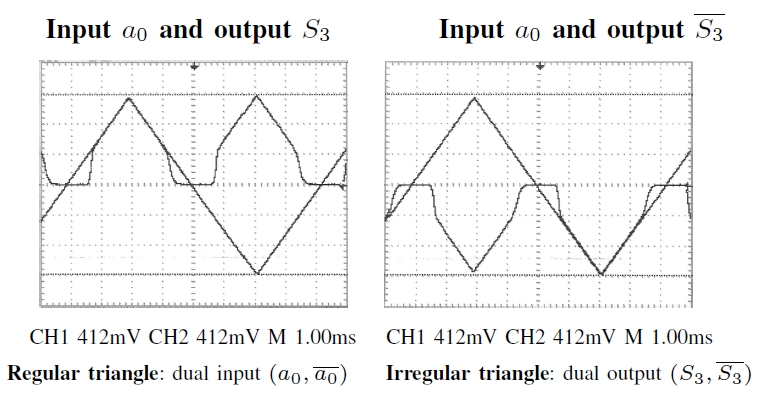Fig. 12.   Oscilloscope snap-view of the 4th dual bits ( S3, S3 ) as a function of the dual switching input bits ( a0, a0 ) = ( X, X ) ,
obtained by direct (adder)
calculation of S = cin + a + b = 1 + 011 X + 0110 = 1 1 X X (with cin = 1 ), measured when using adiabatic pulses.

In Fig.12, the dual output ( S3, S3 ) is given as the example of a constant logic “1“ adiabatic output and compared to the switching input a0. For clarity, the dual signal S3 that is a logic “0“ is also compared to the same input a0 – the complementary a0 being the exact opposite signal of a0 (a0 = - a0).

As previously found in Fig.10, the impact of the threshold voltage can be seen on both dual output signals in Fig.12, at the rise front whereas at the descending one, its impact is superimposed to capacitance effect coming from the probe that slows down the decrease of the signal to the mean valuehere equal to zero, while all the transmission gates of the chip are reaching their open circuit functionality.

Experimental Measurements in backward computation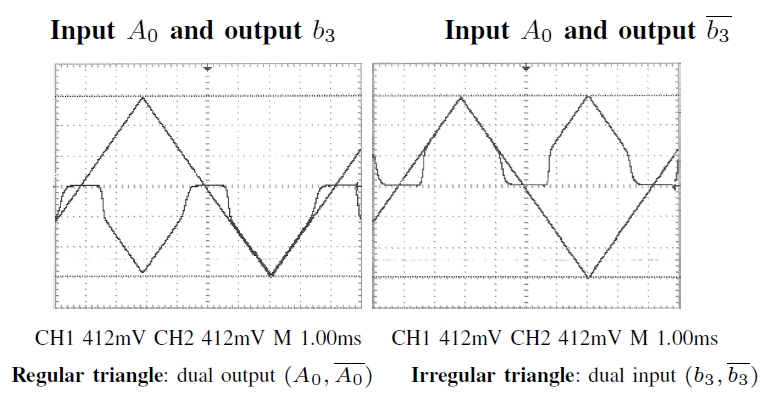Fig. 13.   Oscilloscope snap-view of the 4th dual bits ( b3, b3 ) as a function of the the dual switching bits ( A0, A0 ) = ( X, X ) ,
obtained by reverse
(subtractor) calculation of S - A = b = 0110 - 010 X = 0 0 X X with Cout = 0 and measured when using adiabatic pulses.

As shown in Fig.13, very similar results are obtained when performing a subtraction using the same chip in reverse direction. Here, the output calculated ( b3, b3 ) signal, shown as typical example, is a constant dual zero also compared to the same input signal A0.

As the carry propagates twice through the whole circuit, a maximal delay is obtained between the two extreme output bit pulses (e.g. a0 and b3). The maximum delay τ = 91  μs is measured between outputs a0 and b3 during a subtraction (Fig.14 ). This delay is obtained between two pulses at a frequency 120  Hz, when probes are connected to the outputs. According to these measurements, it is possible to cascade more than 23 Cuccaro gates before the output delay reaches 25 % of the period such that calculation errors may appear.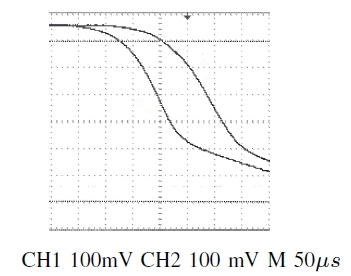Top line : b3, Bottom line : a0.
Fig. 14.   Experimental maximum phase introduced during a computation step.

Cascade Measurement –

Example of switching signal measured on cascaded circuits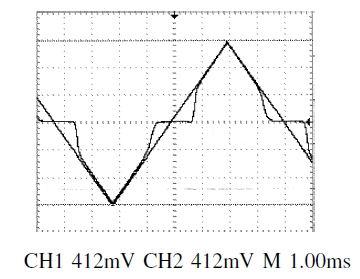class="newline" />
Regular triangle: dual input ( a0, a0 ) , Irregular triangle: dual output ( A0, A0 )

Fig. 15.   Typical adiabatic switch of input and output signal obtained on a cascaded circuit formed by an adder followed by a subtractor
(do-undo computation).

As a last experiment, we cascaded an adder with a subtractor. This experiment allowed to verify that two sequential calculations can be performed and that the addition can really be uncomputed giving back the initial input signal as in the example given in Fig.15.

A large part of the identity truth table (different from the first experiment) has been checked with no errors detected. Also, no significant extra phase nor attenuation occured during this computation steps, giving the indication that a lot of reversible chips may be cascaded.

Nevertheless, the output signal having been transmitted through several transmission gates is not the exact input signal, but a calculated one and inherit of the same characteristics than another calculated output.

VI. Conclusion

We presented real calculations performed in a 350  nm CMOS technology reversible (quantum) adder used in both direction: forward adder and backward subtractor.

The superiority of making use of adiabatic triangle pulses has been demonstrated and explained, showing that using adiabatic pulses on pass-transistor based reversible (quantum) chips is a suitable solution to perform linear calculations.

Acknowledgment

The authors thank the Danish Council for Strategic Research for the support of this work in the frame- work of the MicroPower research project. They are grateful to the Invomec division of Imec v.z.w. (Leuven, Belgium) and the Europractice consortium for the help with the prototyping of the chips.

References

 De Vos A., Lossless computing , Proceedings of the IEEE Workshop on Signal Processing, Pozna ń (2003) pp.7–14.
 Feynman R.P., Quantum mechanical computer , Optics News 11 (1985) pp.11–20.
 Landauer R., Irreversibility and heat generation in the computing process , IBM Journal of Research and Development 5(3), (1961) pp.183–191.
 Bennett C.H., Logical reversibility of computation , IBM Journal of Research and Development 17(6), (1973) pp.525–532.
 Toffoli T., Reversible computing , in: Automata, Languages and Programming, ed. by W. de Bakker, J. van Leeuwen (Springer, Berlin, 1980), Technical Memo MIT/LCS/TM-151, MIT Laboratory for Computer Science (1980) pp.632-644.
 De Vos A., Reversible computer hardware , Electronic Notes in Theoretical Computer Science 253, (2010) pp.17–22.
 Skoneczny M., Van Rentergem Y. and De Vos A., Reversible Fourier transform chip , Proceedings of the 15th International Conference on Mixed Design of Integrated Circuits and Systems (MIXDES 2008), Poznań, June 2008, (2008) pp.281–286.
 De Vos A., Burignat S. and Thomsen M.K., Reversible implementation of a discrete integer linear transformation , Proceedings of the 2nd Reversible Computation Workshop, Bremen (2010) pp.107–110.
 Cuccaro S., Draper T., Moulton D. and Kutin S., A new quantum ripple-carry addition circuit , Proceedings of the 8th Workshop on Quantum Information Processing, Cambridge (June 2005), arXiv:quant-ph/0410184v1 (2004) 9 pages.
 Verdal V., Barenco A. and Ekert A., Quantum networks for elementary arithmetic operations , Physical Review A 54 (1996) pp.147–153.
 Fredkin E. and Toffoli T., Conservative logic , International Journal of Theoretical Physics, 21 (2004) pp.219–253.
 De Vos A., Reversible Computing , WILEY-VCH (2010) 251 pages.
 Van Rentergem Y. and De Vos A., Optimal design of a reversible full adder , International Journal of Unconventional Computing 1 (2004) pp.339-355.